# 1. Introduction

## A little bit of history

The path of a ray of light passing through a gravitational field is bent by the field. This idea is not new; it goes back to Isaac Newton (1643-1727). He thought of light as a flow of "corpuscles", or invisible bodies, which would naturally be affected by a gravitational field. Later in the 18th century, this concept of light was superseded by the wave theory of light, but still attempts were made to calculate the exact deflection angle which would be expected. Soldner in 1801 calculated that this deflection,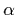, would be given for a point mass M by the equation= 2GM/bc2

where b is the "impact parameter", the closest distance of the ray to the mass, G is the gravitational constant (6.67x10-11Nm2s-2) and c is the speed of light (3x108ms-1). In this equation, the units ofare radians; one radian is equal to about 57 degrees (actually pi radians are exactly equal to 180 degrees).

Albert Einstein returned to the problem in 1916. He had developed a theory of gravity, known as General Relativity, and one of the results he immediately deduced was that the classical result for the deflection angle was wrong by a factor of 2 and should actually be given by= 4GM/bc2

whereis again measured in radians. In the years after Einstein developed General Relativity, this was the easiest of its predictions to test observationally. It was done by Arthur Eddington in 1919, who observed a star near to the sun during a total solar eclipse (the eclipse being necessary to avoid the star's light being swamped by the sun's) and measured that it was displaced in position by a small amount which was closer to Einstein's prediction of 1.75 arcsecond, about 1/2000 of a degree, than to the classical prediction.

 1. The radius of the sun is about 7x108m and its mass is about 2x1030kg. Show that a ray of light which just grazes the Sun and arrives at Earth is deflected by the amount predicted by General Relativity. Answers to questions are at end of this document.

 2. Suppose a ray of light from a distant quasar passes 10kpc from the centre of a galaxy of total mass 1011 that of the sun. (1 kpc = 3x1019m). What is the deflection angle in radians and in arcseconds? What assumptions have you made that may or may not be true?

After its use as a test of General Relativity, there were relatively few advances in the subject for a long time. Einstein himself, though, understood that it might be possible for the bending of light to give very strange effects. In particular, if a background source lay directly behind a foreground mass, its light could be bent around the mass in such a way as to spread the light out into a bright ring. The angular radius of the ring depend on, the deflection angle, and on the relative distance of the source and the lensing object as we shall see in the next section. If the background source were slightly off-centre, more complicated effects could be observed, and in particular multiple images of the background source could be produced.

The layout of such a system - a gravitational lens system - is shown in Figure 1.1. The analogy is with the way a conventional lens forms images, although the mechanism is different; a gravitational lens bends the light by the action of gravity, whereas a conventional lens bends light by refraction at the glass surfaces. The distortions and multiple images produced by gravitational lenses resemble the images produced by looking through the curved bottom of a wine bottle.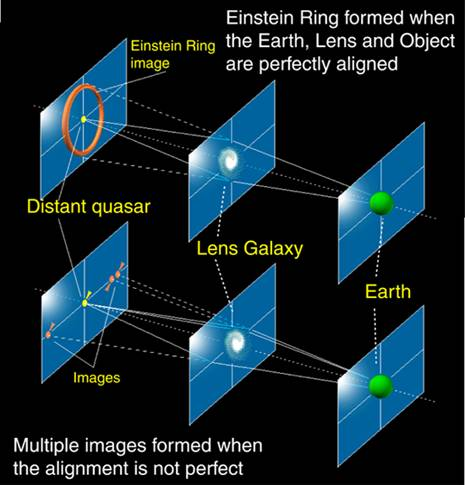Figure 1.1. General arrangement of a gravitational lens system. At the top we have the exactly symmetric case, with the source directly behind the gravitational lens. When the symmetry is not exact (bottom) multiple images of the background source are formed. Note in both cases that light is deflected by the gravitational field of the background source. The deflection angles are small, and they depend on the distance the light ray passes from the centre of the deflector and on the mass distribution of the deflector. This will be dealt with in more detail in section 2.

## Discovery of gravitational lenses

Gravitational lens systems are difficult to discover. A typical gravitational lens system consists of multiple images of a background quasar at vast distances (usually several billions of parcsecs), and in principle all one has to do is to search for this multiple imaging on radio maps or optical plates. Unfortunately, in a typical star-field a gravitational lens is not easy to spot. As the name ("QUasi-StellAR object") implies, quasars at billions of parsecs strongly resemble stars at distances of a few parsecs. For example, Figure 1.2(a) shows the star-field surrounding the first gravitational lens system discovered.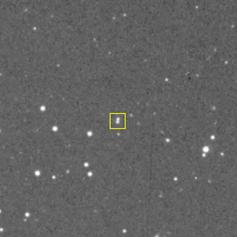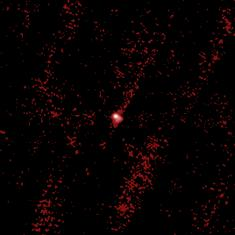Figure 1.2. (a) At left: The star-field around the gravitational lens 0957+561, plotted with 8 arcminutes on a side. The gravitational lens is exactly in the centre and consists of two images of a background quasar 6 arcseconds apart (shown boxed). Without prior knowledge, the system would be very difficult to spot! The task would be even more difficult if the separation of the two images were smaller. (b) At right: The radio image, from the FIRST survey (Becker, White & Helfand, Astrophysical Journal, 450, 559). 0957+561 is a radio source, and is visible in the centre of the field. However, the angular resolution in this picture is slightly worse than the optical, and it is not obvious that we are looking at a gravitational lens. Moreover, the quasar has extended radio structure which cannot be seen without a more detailed radio map (Figure 1.4).

 3. In figure 1.2(b) we see the quasar quite clearly but none of the stars. In other words, radio surveys pick out extremely distant objects and filter out stars in our galaxy. Why do you think this is the case?

The first gravitational lens system was discovered in 1979 by a team led by Dennis Walsh, of the University of Manchester's Jodrell Bank observatory - see the enclosed photocopied article in which Dennis describes the discovery. Throughout the 1960's and early 1970's radio astronomers conducted surveys of the sky (such as the famous 3C survey at Cambridge which led to the discovery of the first quasars) in order to assess what type of objects emitted radio waves. Many of the objects they found were at extremely large distances; when optical plates were searched for coincidences, the radio source often corresponded to a faint galaxy at high redshift.

The work of the Jodrell group particularly focused on finding the sizes of these objects. This could be done using the technique of radio interferometry, in which signals from two telescopes are cobined. See this Layperson's Guide to Radio Astronomy and Interferometry from the MERLIN website at Jodrell Bank for more details.

In the 1960's these experiments used the University of Manchester's Mk1A 250-foot radio telescope (now renamed the Lovell Telescope) together with a smaller, moveable telescope. It turns out that the correlated signal produced when the individual signals from the telescopes are combined falls off as the distance between the telescopes is increased; the smaller the source, the larger the distance needs to be before the correlated signal decreases. The Jodrell Bank group used baselines of over 100km to prove that bright radio sources associated with quasars must be of very small angular size on the sky.

To carry on this work, they needed more surveys of fainter quasars. In the early 1970's they were conducting a survey at 966 MHz using the MkIa, and generated a list of radio sources. However, the single-telescope observations on their own did not give accurate positions, and they used the MkIa telescope together with the newer MkII telescope at the other end of the Jodrell site to form an interferometer. This system gave accurate positions which could in principle be used to examine optical plates and decide which of the objects on the optical plates corresponded to the radio object.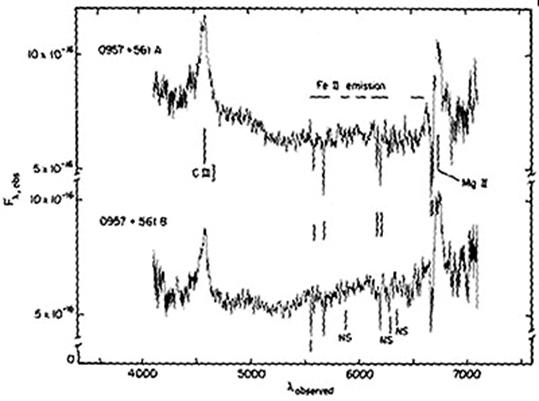Figure 1.3. Optical spectra of the two components of 0957+561 seen in Figure 1.2(a) revealing that they are virtually identical (from Wills & Wills 1980).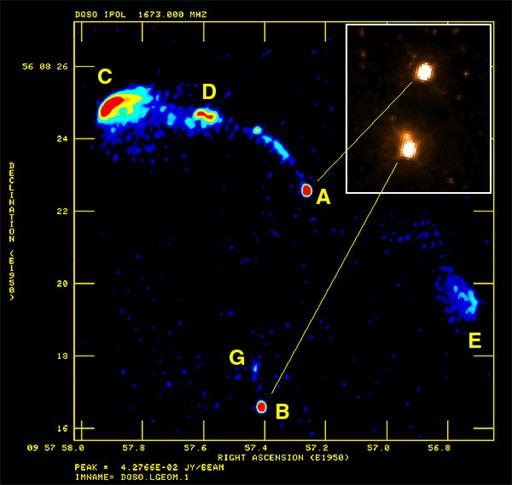Figure 1.4. 0957+561, the first gravitational lens to be discovered. This is a MERLIN radio image. The background source can be seen at the top, and consists of a compact radio core A with ejected radio plasma emerging either side (a jet extending to components C and D on one side and a lobe E on the other). The radio core is imaged into two by the action of the gravitational lens, resulting in the additional component B seen in the image. The inset at top right shows, on a different scale, a Hubble Space Telescope optical image of 0957+561. The two bright components are the double images of the quasar core as seen in Fig. 1.2 and the radio image. The faint blob just above the lower image is emission from the intervening lensing galaxy which can only just be seen in the radio image as component G.

In the next section we will discuss the geometry of lensing systems in more detail.

1. The radius of the sun is about 7x108m and its mass is about 2x1030kg. Show that a ray of light which just grazes the Sun and arrives at Earth is deflected by the amount predicted by General Relativity.

The angle is 1.8 arcseconds.

The mass of the sun is 2x1030kg, so the quantity 4GM/bc2 works out at 8.9x10-6 radians. Converting this to arcseconds involves multiplying by 57 to get to degrees, and then by 3600 to get to arcseconds, giving the final result of 1.8 arcseconds.

2. Suppose a ray of light from a distant quasar passes 10kpc from the centre of a galaxy of total mass 1011 that of the sun. (1 kpc = 3x1019m). What is the deflection angle in radians and in arcseconds? What assumptions have you made that may or may not be true?

Here the mass of the deflector is 2x1041kg, and the impactparameter is 3x1020m. Therefore the quantity 4GM/bc2works out at 1.98x10-6 radians, or about 0.4 arcseconds. Note thatthis calculation has assumed the galaxy is a point mass

3. In figure 1.2(b) we see the quasar quite clearly but none of the stars. In other words, radio surveys pick out extremely distant objects and filter out stars in our galaxy. Why do you think this is the case?